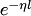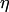# Exponential Weights Algorithms

This is an important family of algorithms in Competitive On-line Prediction. At each trial the weight of each strategy in the benchmark class is multiplied by, whereis a constant called the learning rate andis the strategy's loss. The master's prediction is obtained as a weighted average (in different senses) of the strategies' predictions.

Algorithms in this class include: